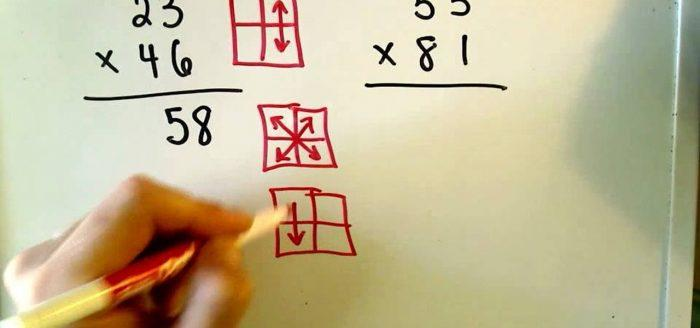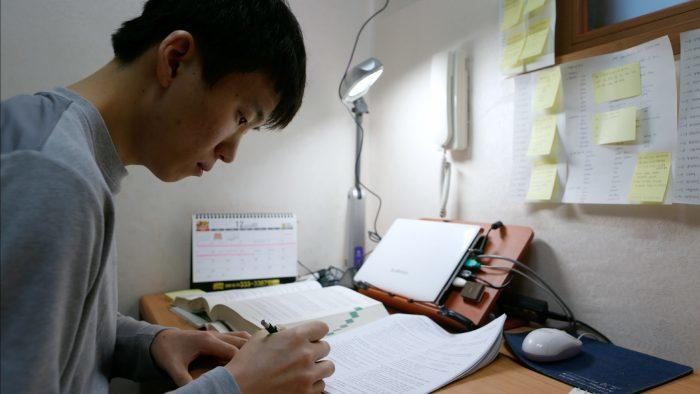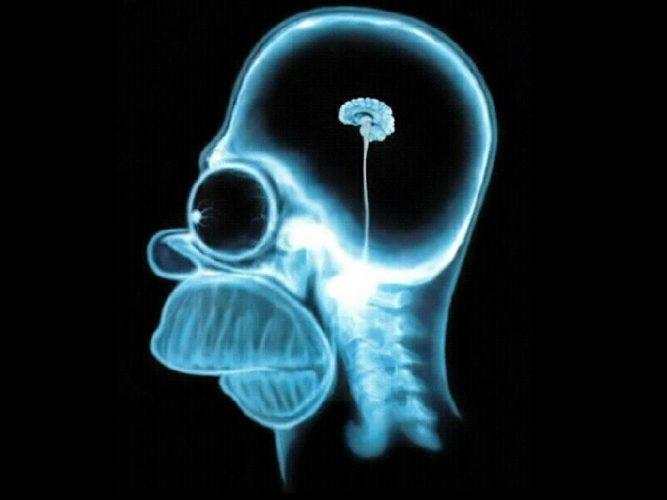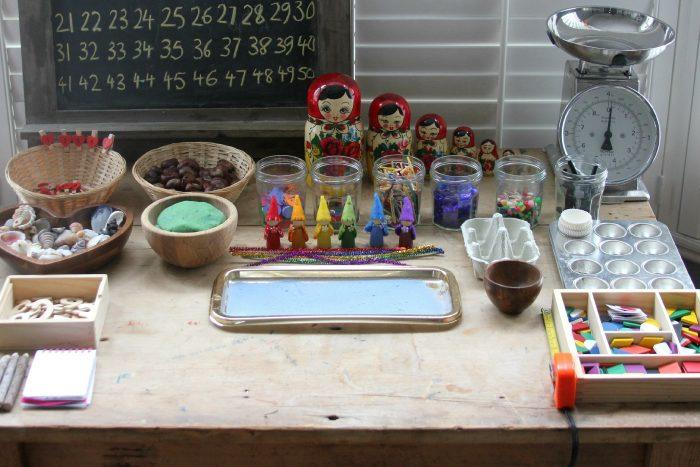Share

# Master the art to improve mental maths

By Jon, published on 31/03/2018 > > > How to Improve Your Mental Maths

India is home to many great Mathematicians; the country has gifted the world with many famous mathematicians over the years, Aryabhata and Ramanujan to name a few. These great men knew the way to master the subject by understanding the premise of the problems. However, students fear mathematics due to all the formulas and the equations used while solving problems and often prefer memorising the solution rather than understanding the problem.

The reason behind this fear is often the age old habit of memorising the equations and formulas rather than understanding and analysing them. Memorising without understanding often leads to fear of forgetting them during the moment of need. Mathematics is a subject that requires logical understanding of the maths tricks, techniques that could make the problem easier to understand and solve.

It is easier to practice maths if the student understands the “how” and “why” of a problem rather than to memorising the one possible solution.You can learn to do sums really quickly, too! (Source: WonderHowTo)

How do you get better at maths? You don’t need to spend hours solving complex problems, all you need is to focus on the basics.

## Getting good grip on mathematics

There are many chapters in mathematics that students find complicated, trigonometry, algebra and arithmetic being the usual suspects and often the most feared chapters. Even though Arithmetic is basic maths, students often commit errors as they try to memorise the solution. This habit of memorising is not the problem, problem is not understanding the basics.

However, improving mental maths can significantly contribute in reducing the stress and fear, turning mathematics from being a problem to one of the most enjoyable subjects. They say: “practice makes a man perfect”, this is true in case of mathematics, as practices improves the rational thinking process, which helps students find logic in the problems. As a student, one must not see Mathematics as rocket science, but as a logical mental exercise. Mental arithmetic practice can ease the pressure on students trying to gain control over the subject.

Back to basics is usually the first step, one must go back to BODMAS, addition, subtraction, multiplication, and division tables, and learn the square roots (up to 12 or 15) to start off with.All learning begins with the basics, even maths! (Source: TheConversation)

## Improving your mental math skills

For children, basic mathematics seems like a mountain task, for students in higher classes geometry, algebra seems difficult, as finding the correct solution is often linked with better marks in exams. This search for solutions and tricks leads to an unknown fear inside their mind. Due to fear, they avoid using mental maths and prefer calculators even for basic calculations.

Mental maths practice often improves logical thinking, the numbers become like a language easy to remember and solve. Technology has made calculations easier, which ensures our life is not complicated while solving problems. However, we tend to become over-dependent on it, and look for one when the situation can be resolved through mental calculations. As a student, one must not depend on technology for simple solutions. This is where mental maths sharpens our logical awareness and helps us make better decisions.

You can start with simple basic mental calculations, like calculating the bill of your groceries or your food bill in a restaurant.With a good teacher you’ll quickly learn to do maths without even thinking! (Source: IDN Times)

## The process of practicing mathematics mentally

One should know the process of approaching basic mathematics so that complex calculations can be resolved easily. For example, it is best to recapitulate and try to improve the capability of remembering simple mathematical tables like addition, division or the square roots up to 10 or 15 and the multiplications.  They all follow an analytical technique and with some simple tips and tricks one can improve the capability of the thought process and get the right solution.

Say we need to multiply 25 x 24. The best way is to multiply 25 x 20 and add 100 to it. Again for adding 8 with 27, we can break the number like 27+3 = 30+ 5 = 35. There is no need for calculations with the fingers or be in tension and start biting the ends of the pencil or the pen for the solutions. Practicing tips for regular problems contributes not only solving complex problems but also helps in daily life.Are you a maths whizz? (Source: IdleWords)

Our brain and hands need to adapt and activate its various faculties.  This is how you understand to calculate quickly both in your private maths classes and in your daily life.

Three simple steps to improve:

1. Understand
2. Revise
3. Practice

Follow this regularly, especially in areas where equations and formulas are used more often, understanding the problems is like the stepping stone for mental maths.

## The need for mental mathematics

Don’t swim before you can walk. The same has to be done for maths. You can learn maths in many different ways so don’t waste your time with tricks that you don’t understand.

The world as a whole has changed considerably over time due to technological growth and invention of gadgets. Many of these gadgets are reducing our potential of logical thinking or doing simple work, including subjects that are being taught to the students. Students are relying more on gadgets forgetting the ability of their brain and its capability to solve problems.

If one utilises the brain in the right manner, logical reasoning for even difficult problems can be achieved without much brainstorming. As one improves their logical and rational thinking process, the brain becomes active, otherwise it stays under-utilised due the gadgets in hand. Developing the right thought process, and justifying the correct reasoning, considerably eases and simplifies student life helping to solve mathematics problems mentally.

Improve your knowledge with regular maths exercises like Sudoku and keep practicing.It’s definitely more haste, less speed when it comes to maths. (Source: CreativINDIE)

The principle behind mastering the art

A good teacher is all you need, one who can guide through the process and techniques of mental maths, understanding the right way to solve problems helps develop logic. Logic helps identifying a pattern, this improves with time, as the student practices more, this logic becomes a base for future problems. This base of logic helps not only in understanding the subject but also reduces the fear of mathematics.

Human brains work the same as the computer. Contrarily we can say, the computers are replicas of human brains. The capacity of our brain is much more than any simple computer and uses less energy than the machines to solve mathematical problems. If we consider the brain as the core part of the hard drive in a computer, the knowledge, and the skills are like the operating system. More we hone them up; the brain becomes more efficient, giving the right solution. As one enters into various stages of life, the mental process of thinking and solving mathematics problems evolves along with each step.How do you improve the best recipe? Add a sprinkle of maths, of course! (Source: The Imagination Tree)

## Take your time to learn

Mathematics can be learnt in various ways. There is never one way to solve a problem, there are multiple shortcuts, but one should always avoid taking them if they do not understand the logic.

Watching online videos and clarifying doubts can improve the learning skills to a great extent for staying ahead of others in the maths class getting the answers promptly. Once self-confidence is boosted up, no mathematical problems will look tough. The conquerors of the Mount Everest had practiced climbing other peaks before completing the most challenging summit of the world. Nothing is impossible if each step is correctly thought, and increasing the level of hardness, and clearing the doubts from a right teacher or joining a good mathematics teacher is the process of sharpening the brain and getting better for mastering the subject.

### Improve concentration

There are many ways to improve concentration:

1. Yoga: Yoga and meditation has proven to increase physical fitness and concentration levels. Any student can do both to increase the levels and focus the brain properly and think logically getting the correct mental reasoning for maths solutions.
2. Sports: Physical workout increases mental awareness. Mental awareness improves reasoning, which in return improves thinking process.
3. Puzzles: Play games such as Sudoku, which requires logic and understanding.

The students need to discard the fear of mathematics. One should always look for avenues and find out how to improve mental arithmetic and go ahead with the process. It is not a tough task but to activate the brain to think correctly to get to the ultimate solution.  One should feed the brain to get the desired outcome. No matter how much complex may be the arithmetic, if the basics are correctly thought and inscribed in the brain, the solutions come easy and faster. It is time to be ahead of others practicing mental mathematics.

Whether you’re working with a teacher or not, all you need is to train your brain and work on your mathematical reflexes.

Share(1 votes, average: 5.00 out of 5)Loading...Recent comment authors